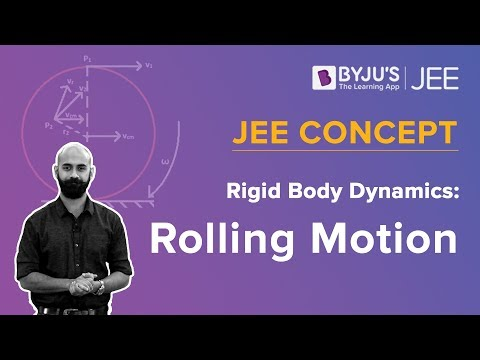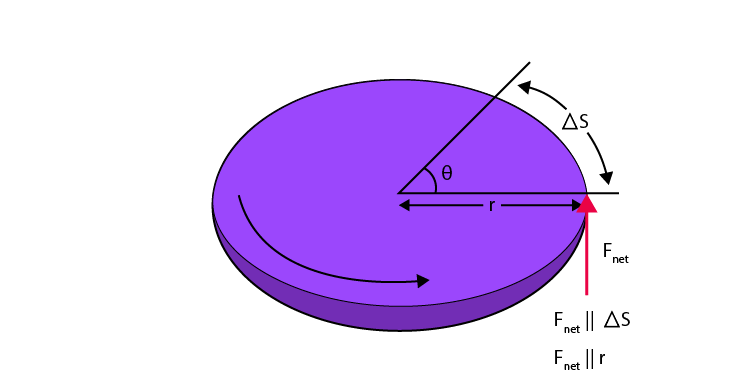# Rotational Kinetic Energy

The kinetic energy of a rotating body can be compared to the linear kinetic energy and described in terms of the angular velocity. The extended object’s complete kinetic energy is described as the sum of the translational kinetic energy of the centre of mass and rotational kinetic energy of the centre of mass. The rotational kinetic energy is represented in the following manner for a constant axis of rotation.

## What is Rotational Kinetic Energy?

Rotational energy occurs due to the object’s rotation and is a part of its total kinetic energy. If the rotational energy is considered separately across an object’s axis of rotation, the moment of inertia is observed. Rotational energy also known as angular kinetic energy is defined as:

The kinetic energy due to the rotation of an object and is part of its total kinetic energy.

Rotational kinetic energy is directly proportional to the rotational inertia and the square of the magnitude of the angular velocity. A rolling object has both translational and rotational kinetic energy.

## Rotational Kinetic Energy Formula

The rotational kinetic energy of a rotating object can be expressed as half of the product of the angular velocity of the object and moment of inertia around the axis of rotation. Mathematically written as:

 $$\begin{array}{l}K_R = \frac{1}{2}I ω^2\end{array}$$

Where,

• KR is Rotational Kinetic energy
• I is the moment of inertia
• ω is the angular velocity

The expression can be designed for linear and rotational kinetic energy parallelly from the principle of work and energy. Consider the particular parallel between a fixed exerted torque on a wheel with a moment of inertia and force on a mass m and both beginning from the rest.

According to Newton’s second law, the acceleration is equivalent to the resultant velocity divided by the time and average velocity is half of the final velocity. In rotational case, the angular acceleration given to the wheel is fetched from Newton’s second law of rotation.

The mechanical work that is required during rotation is the number of torque of the rotation angle. The axis of rotation for unattached objects is mostly around its centre of mass.

## Rotational Kinetic Energy Dimensional Formula

We know that rotational kinetic energy is given as:

$$\begin{array}{l}K_R = \frac{1}{2}I ω^2\end{array}$$

Rotational kinetic energy

$$\begin{array}{l}K_R = \frac{1}{2}\,\, \textup{[Moment of inertia ×}\,\, {\textup{(Angular velocity)}}^2]\end{array}$$

Dimensional formula of moment of inertia = M1L2T0

Dimensional formula of angular velocity = M0L0T-1

By substituting the dimensional formula of moment of inertia and angular velocity, we get,

Dimensional formula of rotational kinetic energy = M1L2T-2

## Unit of Rotational Kinetic Energy

Following is the table with unit of rotational kinetic energy in SI and MKS system:

 SI unit system Joules (J) MKS unit system kg.m2.s-2Please note the relationship between the result of rotational energy and the energy held by linear motion.

 $$\begin{array}{l}K_{Transitional} = \frac{1}{2}mv^2\end{array}$$

The moment of inertia plays the role of the mass, and angular velocity plays the role of linear velocity v in a rotating system.

You may also want to check out these topics given below!

### Rotational Power Formula

Power for rotational systems depends upon torque and frequency of the rotation. By knowing the values of torque and revolutions per minute, power can be calculated. Following is table explaining the formula for rotational power:

 $$\begin{array}{l}P=\frac{\tau \times\frac{2}{\pi}\times rpm}{60}\end{array}$$

Where,

• P is the rotational power
• τ is the torque
• rpm is the revolutions per minute

## Rotational Kinetic Energy DerivationFor any object to rotate such as grindstone or merry-go-rounds, work must be done. Consider a situation where work is done resulting in a rotational situation such that the net force exerted is perpendicular to the radius of the disk and continues to remain perpendicular as the disk starts to rotate. The net work done is the product of force times the arc length travelled since force is parallel to the displacement which is given as:

net W = (net F)s

By multiplying and dividing the above equation with r, we get quantities related to rotational quantities:

$$\begin{array}{l}\textup{net W = (r net F) }\frac{s}{r}\end{array}$$

From the above equation, we understand that,

$$\begin{array}{l}\textup{r net F = net and }\Delta \frac{s}{r} = θ\end{array}$$

Therefore, net W = (net) θ

The above equation is the expression for rotational work.

To obtain an expression for rotational kinetic energy, let net = Iα

Therefore, net W = I

The net work done is given as:

(net F) Δs [since net force on the disk is perpendicular to the radius of the disk as the disk is rotating because of the force]

From rotational kinematics equation , we get

$$\begin{array}{l}\omega ^{2}=\omega _{0}^{2}+2\end{array}$$
$$\begin{array}{l}\textup{net W =}\,\, \frac{1}{2}I\omega ^{2}-\frac{1}{2}I\omega _{0}^{2}\end{array}$$

The above equation is the work-energy theorem for rotational motion.

We can define 1/2(Iω2) to be rotational kinetic energy for an object with the moment of inertia I and angular velocity ω from the analogy with translational motion.

Therefore, rotational kinetic energy is,

$$\begin{array}{l}K_R = \frac{1}{2}I ω^2\end{array}$$

## Rotational Kinetic Energy Example Problems

Q1. A round grindstone with a moment of inertia 1600 kg.m2 is rotating at an angular velocity of 6 radians.s-1. What is grindstone’s rotational kinetic energy?
Given:
Moment of inertia, I = 1600 kg.m2
Angular velocity, w = 6 radians.s-1
From rotational kinetic energy formula,

$$\begin{array}{l}K_R = \frac{1}{2}I ω^2\end{array}$$
$$\begin{array}{l}K_R = \frac{1}{2}(1600\;kg.m^{2})(6\;radians.s^{-1})^{2}\end{array}$$
$$\begin{array}{l}K_R = \frac{1}{2}(1600\;kg.m^{2})(36\;s^{-2})\end{array}$$
$$\begin{array}{l}K_R = \frac{1}{2}(57600\;kg.m^{2}.s^{-2})\end{array}$$

K= 28800 kg.m2.s-2

KR = 28800 J

Therefore, the rotational kinetic energy of the grindstone is 28800 J.

## Frequently Asked Questions – FAQs

Q1

True.
Q2

### What is the formula to calculate rotational kinetic energy formula?

$$\begin{array}{l}K_{R}=\frac{1}{2}I\omega^{2}\end{array}$$
Where,
• KR is Rotational Kinetic energy
• I is the moment of inertia
• ω is the angular velocity
• Q3

### What is the formula to calculate dimensional formula of rotational kinetic energy?

The dimensional formula of rotational kinetic energy is M1L2T-2.
Q4

### What is the SI unit of rotational kinetic energy?

The SI unit of rotational kinetic energy is Joules (J).
Q5

### What is the rotational power formula?

The rotational power formula is given by:
$$\begin{array}{l}P=\frac{\tau \times\frac{2}{\pi}\times rpm}{60}\end{array}$$
Test your knowledge on Rotational Kinetic Energy